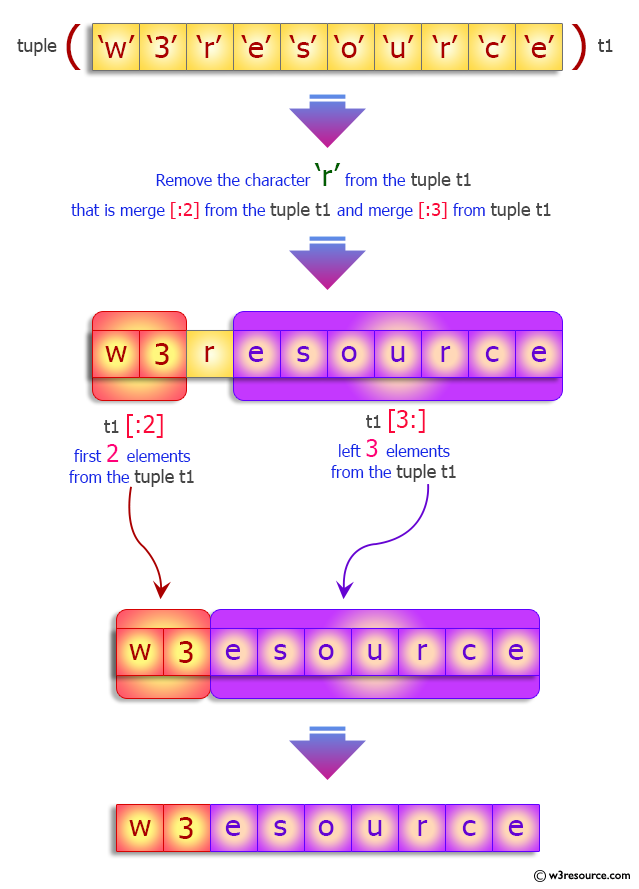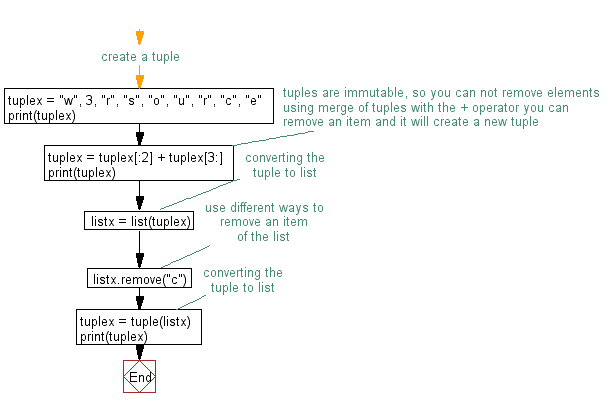﻿ Python: Remove an item from a tuple - w3resource# Python Exercise: Remove an item from a tuple

## Python tuple: Exercise-12 with Solution

Write a Python program to remove an item from a tuple.

Sample Solution:-

Python Code:

``````#create a tuple
tuplex = "w", 3, "r", "s", "o", "u", "r", "c", "e"
print(tuplex)
#tuples are immutable, so you can not remove elements
#using merge of tuples with the + operator you can remove an item and it will create a new tuple
tuplex = tuplex[:2] + tuplex[3:]
print(tuplex)
#converting the tuple to list
listx = list(tuplex)
#use different ways to remove an item of the list
listx.remove("c")
#converting the tuple to list
tuplex = tuple(listx)
print(tuplex)
```
```

Sample Output:

```('w', 3, 'r', 's', 'o', 'u', 'r', 'c', 'e')
('w', 3, 's', 'o', 'u', 'r', 'c', 'e')
('w', 3, 's', 'o', 'u', 'r', 'e')
```

Pictorial Presentation:Flowchart:## Visualize Python code execution:

The following tool visualize what the computer is doing step-by-step as it executes the said program:

Python Code Editor:

Have another way to solve this solution? Contribute your code (and comments) through Disqus.

What is the difficulty level of this exercise?

Test your Programming skills with w3resource's quiz.

﻿

## Python: Tips of the Day

Clamps num within the inclusive range specified by the boundary values x and y:

Example:

```def tips_clamp_num(num,x,y):
return max(min(num, max(x, y)), min(x, y))
print(tips_clamp_num(2, 4, 6))
print(tips_clamp_num(1, -1, -6))
```

Output:

```4
-1
```